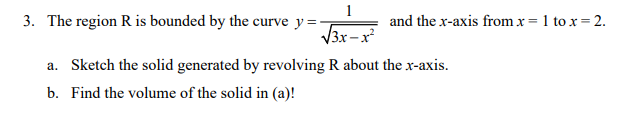### Create an Account

Already have account?

### Forgot Your Password ?

Home / Questions / 1 3. The region R is bounded by the curve y=- and the x-axis from x= 1 tox=2. 13x-r? a. Sk...

# 1 3. The region R is bounded by the curve y=- and the x-axis from x= 1 tox=2. 13x-r? a. Sketch the solid generated by revolving R about the x-axis. b. Find the volume of the solid in (a)!

1 3. The region R is bounded by the curve y=- and the x-axis from x= 1 tox=2. 13x-r? a. Sketch the solid generated by revolving R about the x-axis. b. Find the volume of the solid in (a)!Apr 13 2021 View more View Less

#### Answer (Solved)Subscribe To Get Solution